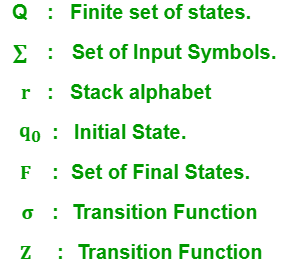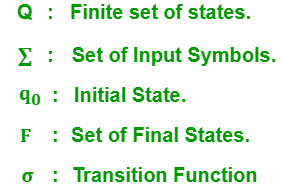Related Articles
Difference between Pushdown Automata and Finite Automata
• Last Updated : 23 Dec, 2019

Pushdown Automata:
A Pushdown automata (PDA) is a finite state machine with an added stack storage. Additional stack is used in making the decision for transitions apart from input symbols and current state. It contains the following 7 tuples:Finite Automata:
A Finite Automata is a mathematical model of any machine by which we can calculate the transition of states on every input symbol.Each transition in finite automata depends on the input symbols and current transition state. It contains the following 5 tuples:Let’s see the difference between Pushdown Automata and Finite Automata:

S.NO Pushdown automata finite automata
1. For Type-2 grammar we can design pushdown automata. For Type-3 grammar we can design finite automata.
2. Non – Deterministic pushdown automata has more powerful than Deterministic pushdown automata. Non-Deterministic Finite Automata has same powers as in Deterministic Finite Automata.
3. Not every Non-Deterministic pushdown automata is transformed into its equivalent Deterministic pushdown Automata . Every Non-Deterministic Finite Automata is transformed into an equivalent Deterministic Finite Automata
4. Context free languages can be recognized by pushdown automata. Regular languages can be recognized by finite automata.
5. Pushdown automata has the additional stack for storing long sequence of alphabets. Finite Automata doesn’t has any space to store input alphabets.
6. It gives acceptance of input alphabhets by going up to empty stack and final states. It accepts the input alphabets by going up to final states.

Attention reader! Don’t stop learning now. Get hold of all the important CS Theory concepts for SDE interviews with the CS Theory Course at a student-friendly price and become industry ready.

My Personal Notes arrow_drop_up
Recommended Articles
Page :